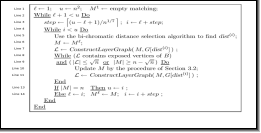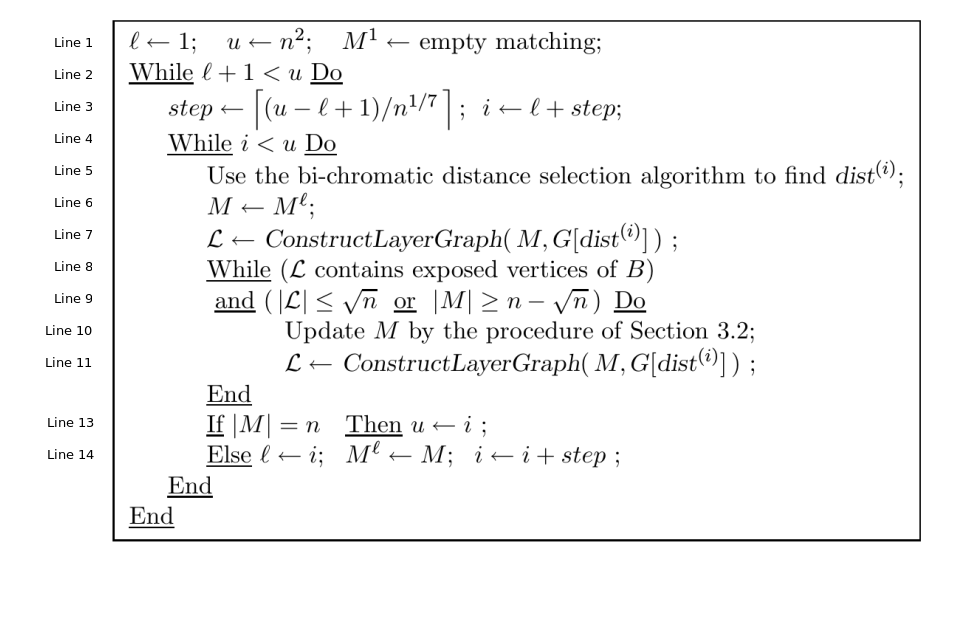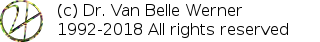Home Papers Reports Projects Code Fragments Dissertations Presentations Posters Proposals Lectures given Course notes# On the impossibility of implementing the O(n^1.5) bottleneck distance matching

Werner Van Belle1* - werner@yellowcouch.org, werner.van.belle@gmail.com

1- Yellowcouch;

Abstract :  Recently we tried implementing 'Geometry helps in Bottelneck Matching and Related Problems'. In doing so we figured out that the presented algorithm does not work.

Keywords:  bottleneck distance, bipartite matching
Reference:  Werner Van Belle; On the impossibility of implementing the O(n^1.5) bottleneck distance matching; Computer Science; YellowCouch; January 2017

Recently, we tried implementing a bottleneck matching, as described in the paper Geometry Helps in Bottleneck Matching and Related Problems by Alon Efrat, Alon Itai and Matthew J. Katz . To do so, we created representations for vertices, layered graphs and matchings. We then wrote code to compute augumented paths, which in general bipartite graphs has a O(n^2.5) performance .

The authors then aim to improve these results by taking the geometry of the problem into account. This is provided through a 'dist oracle', which allows the layered graph to quickly find neighboring vertices. This approach is then worked out for a number of scenarios. The best, and most cited, result of their paper is an O(n^1.5) performance for bottleneck matching in the euclidean planar case, described in section 5 of their paper. The resulting algorithm, given on page 15 (presented below) does however not work. To demonstrate that, we will give two counter examples.In our first counterexample, we work with 2 datasets of 3 points (n=3). At line 1 of the algorithm we have l=1, u=9. At line 3 of the algorithm we have a real step value of 7.692. Its ceiling is 8, thus step=8 and i=9. The inner loop at line 4 is immediatelly skipped because i<u (9<9 is false). At line 2, we start another cycle of the outerloop by verifying that l+1<u (1+1<9), which brings us back to line 3, where we are in exactly the same state as with the previous iteration. With 3 values, irrespective of what they are, the algorithm hangs indefinitely.

It is worth providing a second counterexample, one in which the inner loop is accessed but for which the algorithm will not generate a maximal matching. We start with 4 points for each set

 Set A Set B Point ID Coordinates Point ID Coordinates 1 (58,8) 2 (87,21) 3 (13,94) 4 (54,62) 5 (86,5) 6 (29,70) 7 (4,55) 8 (2,7)

At line 1, we set l=1, u=16 and M^1={}
At line 2, we enter the outerloop (2<16)
At line 3, we calculate the stepsize 13.125365696122207 and obtain its ceiling. step=14. Thus, i=15
At line 4, 15<16, we thus go to line 5
At line 5, we must find dist(i), which is, the i'th largest value from the multiset {dist(a,b)|a in A, b in B}. At this point we computed all pairwise distances and sorted them.

```dist(16) = 16.0312195418814
dist(15) = 28.844410203711913
dist(14) = 29.154759474226502
dist(13) = 31.78049716414141
dist(12) = 48.041648597857254
dist(11) = 50.48762224545735
dist(10) = 52.009614495783374
dist(9) = 54.147945482723536
dist(8) = 56.00892785976178
dist(7) = 65.36818798161687
dist(6) = 68.4470598345904
dist(5) = 84.02380615040002
dist(4) = 86.45229898620394
dist(3) = 87.69264507357501
dist(2) = 89.69392398596462
dist(1) = 103.9471019317037
```

Thus dist(15)=28.844410203711913.
At line 6, The current matching M is set to M^1, which was empty. M={}.
At line 7, we create the initial layered graph. It contains 2 layers. The first layer are all exposed vertices (which, given the currently empty matching, are all points from A).

```1:(58,8)
3:(13,94)
5:(86,5)
7:(4,55)```

The second layer are all vertices of B that are within range of any vertex from layer 1. In our case these are point numbers 2 and 6.

```2:(87,21)
6:(29,70)```

At line 8 we find that the layered graph does contain exposed vertices of B and at line 9 we find that the number of layers we have so far (2) is smaller than the square root of 16, so we go into the innermost loop.
At line 10, we update the matching by finding an appropriate augumenting path. This leads to a new matching M:

```    3:(13,94) ~ 6:(29,70) distance between them: 28.844410203711913
5:(86,5)  ~ 2:(87,21) distance between them: 16.0312195418814```

At line 11, we compute a new layered graph given the connections from M. The new L is empty because no exposed vertices of B are within range 28.844410203711913. That makes us leave the innermost while loop. At line 13, we check the cardinality of matching M to decide on how to progress to the next step. |M|=2, therefore we update the variables according to line 14: l=i=15, M^15=M and i=i+step=29.
At line 4 we then find that i is not smaller than u (29>16), thus we leave to the outer loop.
At line 2 we find that l+1 is not less than u. With that, the algorithm finishes with a matching that is only 2 elements large; and thus not maximal.

The best matching, which we computed by creating all possible maximal matchings and measuring their bottleneck distance, is 54.15 as follows:

``` 1:(58,8) <-> 4:(54,62) d:54.147945482723536
3:(13,94) <-> 6:(29,70) d:28.844410203711913
5:(86,5) <-> 2:(87,21) d:16.0312195418814
7:(4,55) <-> 8:(2,7) d:48.041648597857254```

References

 1 Geometry Helps in Bottleneck Matching and Related Problems Alon Efrat, Alon Itai, Matthew J. Katz ALGORITHMICA, January 2000 2 An n^{2.5} algorithm for maximum matchings in Bipartite Graphs John Hopcroft, Richard M. Karp SIAM J. Comput. Vol 2, No 4, December 1973

 http://werner.yellowcouch.org/werner@yellowcouch.org